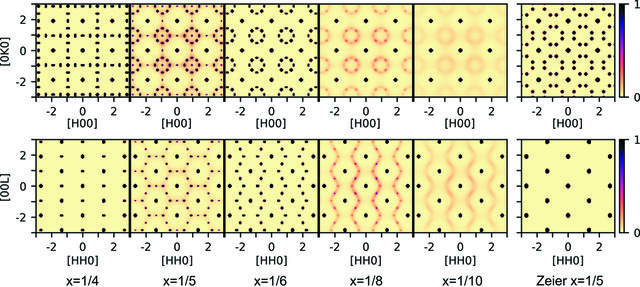disable zoom     view article Figure 5 Calculated scattering for the ground states at different compositions in the HK0 (upper row) and HHL planes (lower row). For all these models the scattering has been averaged to the symmetry of the average structure to simulate the expected measurement for a sample with multiple domains. The scattering for x = 1/4, x = 1/6 as well as the x = 1/5 structure suggested by Zeier et al. (2017) are calculated from the ordered vacancy structures shown in Fig. 3. For the x = 1/5, x = 1/8 and x = 1/10 systems the scattering is calculated from the Monte Carlo simulated structures. The calculated scattering for several other possible ground-state vacancy structures and the scattering in non-symmetry averaged planes are shown in the Supporting information.

IUCrJ
Volume 7| Part 4| July 2020| Pages 673-680
ISSN: 2052-2525